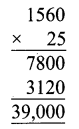Samacheer Kalvi 6th Maths Solutions Term 1 Chapter 1 Numbers Ex 1.3

Tamilnadu Samacheer Kalvi 6th Maths Solutions Term 1 Chapter 1 Numbers Ex 1.3Question 1.
Fill in the blanks.

1. If Arulmozhi saves ₹ 12 per day then she saves ₹ ____ in 30 days.
2. If a person ‘A’ earns ₹ 1800 in 12 days, then he earns ₹ ____ in a day.
3. 45 ÷ (7 + 8) – 2 = _____

Solution:

1. 12 × 30 = ₹ 360
2. $$\frac{1800}{12}=150$$
3. 45 ÷ 15 – 2 = 3 – 2 = 1

Question 2.
Say True or False.

1. 3 + 9 × 8 = 96
2. 7 × 20 – 4 = 136
3. 40 + (56 – 6) ÷ 2 = 45

Solution:

1. False
2. True
3. False

Question 3.
The number of people who visited the Public Library for the past 5 months was 1200, 2000, 2450, 3060 and 3200 respectively. How many people visited the library in the last 5 months.
Solution:
People visited the library for past 5 months = 1200 + 2000 + 2450 + 3060 + 3200 = 11910
Total people visited = 11910

Question 4.
Cheran had a bank savings of Rs 7,50,250. He withdrew Rs 5,34,500 for educational purpose. Find the balance amount in his account.
Solution:
Savings = Rs 7,50,250 Cash withdrawn = Rs 5,34,500
Balance amount = Rs 7,50,250 – Rs 5,34,500 = Rs 2,15,750Question 5.
In a cycle factory, 1560 bicycles were manufactured every day. Find the number of bicycles manufactured in 25 days.
Solution:
Number of bicycles manufactured in one day = 1560
Number of bicycles manufactured in 25 days = 1560 × 25 = 39000Question 6.
Rs 62500 was equally distributed as a New Year bonus for 25 employees of a company. How much did each receive?
Ans:
Total amount = Rs 62500
Total number of employees = 25
Bonus amount received by each employee = Rs 62500 ÷ 25
= RS $$\frac{62500}{25}$$
= Rs 2500

Question 7.
Simplify the following numerical expression:
(i) (10 + 17) ÷ 3
(ii) 12 – [3 – {6 – (5 – 1)}]
(iii) 100 + 8 ÷ 2 + {(3 × 2) – 6 ÷ 2}
Solution:
(i) (10 + 17) ÷ 3 (Given)
= 27 ÷ 3 (Bracket completed first)
= 9 (÷ completed)
∴ (10 + 17) ÷ 3 = 9

(ii) 12 – [3 – {6 – (5 – 1)}] (Given)
= 12 – [3 – {6 – 4}] (Innermost bracket completed first)
= 12 – [3 – 2] (Again Inner bracket completed second)
= 12 – 1 (Bracket completed third)
= 11 (- completed)
∴ 12 – [3 – {6 – (5 – 1)}] = 11

(iii) 100 + 8 ÷ 2 + {(3 × 2) – 6 ÷ 2} (Given)
= 100 + 8 ÷ 2 + {6 – 6 ÷ 2} (Innermost bracket completed first)
= 100 + 8 ÷ 2 + {6 – 3} (To remove the next bracket ÷ within the bar completed second)
= 100 + 8 ÷ 2 + 3 (bar completed third)
= 100 + 4 + 3 (÷ completed fourth)
= 107 (+ completed)
∴ 100 + 8 ÷ 2 + {(3 × 2) – 6 ÷ 2} = 107Objective Type Questions

Question 8.
The value of 3 + 5 – 7 × 1 is …….
(a) 5
(b) 7
(c) 8
(d) 1
Solution:
(d) 1

Question 9.
The value of 24 ÷ {8 – (3 × 2)} is ______
(a) 0
(b) 12
(c) 3
(d) 4
(b) 12
24 ÷ {8 – 3 × 2} = 24 ÷ {8 – 6} = 24 ÷ 2 = 12

Question 10.
Use BIDMAS and put the correct operator in the box.
2₹6 – 12 ÷ (4 + 2) = 10
(a) +
(b) –
(c) ×
(d) ÷
Solution:
(c) ×